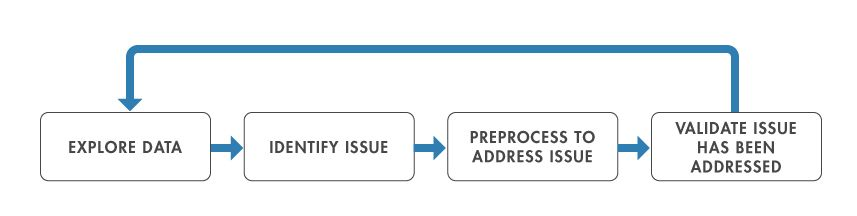# Speeding Up Data Preprocessing for Machine Learning

Chapter 1: Exploring Data

Chapter 1

## Exploring Data

This ebook demonstrates the tasks involved to preprocess data used for machine learning algorithms in MATLAB®.

If you have worked with machine learning, you know that you must preprocess the data. You also know that this can be a tedious process when handled manually. You need to be able to make updates to preprocessing scripts within a framework that allows you to quickly evaluate the impact of changes on the accuracy of the machine learning model.

If you think back to early math classes, there were clear rules on the order of operations (PEMDAS), remembered with mnemonics like “Please Excuse My Dear Aunt Sally.” Whether your type of problem concerns apples or mortgage rates, you know that 2 * (6+4)2 = 200.

The order of operations for data preprocessing tasks is not so straightforward. There are few hard and fast rules when it comes to what order you should do tasks, but every problem has its own factors that may affect what comes first.

Before data can be preprocessed, you need to know which preprocessing tasks are needed. Querying, visualizing, and otherwise exploring your data will provide insight into where you should focus your effort and lead to an iterative workflow in which exploration informs preprocessing. Repeat this process until exploration no longer yields issues that need to be addressed.To keep track of the iterations through this workflow, it is helpful to keep track of preprocessing operations in a script. This also makes it easier to adjust the order of your preprocessing steps, as the resulting preprocessed data may vary depending on the order in which preprocessing steps were applied.

Let’s look at an example MATLAB script for preprocessing data.## Create Data That Needs Preprocessing

```% Create a sine wave with noise
% and remove some of the values
% so they're missing.

rng default;
t = 0:0.01:5;
y = sin(t) + randn(size(t));
missingIdx = randi([1 length(y)],100,1);
y(missingIdx) = NaN;

% Plot the data. You can see gaps
% in the plot that represent the
% missing data.

figure;
plot(t,y)
```## Preprocess Missing Values

```% Use the fillmissing function
% with linear interpolation to
% fill the gaps in the data.

y_filled = fillmissing(y, "linear");

% Add the filled data to the
% plot. You can see that the
% gaps are now filled.

hold on;
plot(t, y_filled, ':r')
```## Clean Missing Data with a Live Editor Task

Alternatively, you could use a Live Editor task to perform this preprocessing. Live Editor tasks can be found in the MATLAB toolbar under the Live Editor > Task drop-down. The Clean Missing Data Live Editor task shows a plot of the cleaned data and missing values that were filled. It also presents the various methods that can be used to clean the data.## Smooth Data

Next you can use the Smooth Data Live Editor task to smooth the noise. Apply a Gaussian filter with a moving window of width 1.

In this script, the missing data was cleaned before the smoothing was applied. But this might not always be the best choice. Because all of the steps are captured in the script, it would be easy to go back and move sections around to see what effect changing the preprocessing order has on the results.